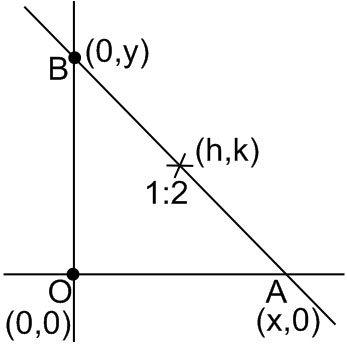# A stair-case of length l rests against a vertical wall and a floor of a room,. Let P be a point on the stair-case, nearer to its end on the wall, that divides its length in the ratio 1 : 2. If the stair-case begins to slide on the floor, then the locus of P is : Option 1) an ellipse of eccentricityOption 2) an ellipse of eccentricityOption 3) a circle of radiusOption 4) a circle of radiusAs we learnt in

Selection formula -

- wherein

If P(x,y) divides the line joining A(x1,y1) and B(x2,y2) in ration

and

Eccentricity -

- wherein

For the ellipseWe know length = l

so, x2, + y2 = l2

now using section formula

P(h, k) is

x = 3h;

, an ellipse

so

Option 1)

an ellipse of eccentricitythis is incorrect

Option 2)

an ellipse of eccentricitythis is correct

Option 3)

a circle of radiusthis is incorrect

Option 4)

a circle of radiusthis is incorrect

### Preparation Products

##### JEE Main Rank Booster 2021

This course will help student to be better prepared and study in the right direction for JEE Main..

₹ 13999/- ₹ 9999/-
##### Knockout JEE Main April 2021 (Subscription)

An exhaustive E-learning program for the complete preparation of JEE Main..

₹ 4999/-
##### Knockout JEE Main April 2021

An exhaustive E-learning program for the complete preparation of JEE Main..

₹ 22999/- ₹ 14999/-
##### Knockout JEE Main April 2022

An exhaustive E-learning program for the complete preparation of JEE Main..

₹ 34999/- ₹ 24999/-